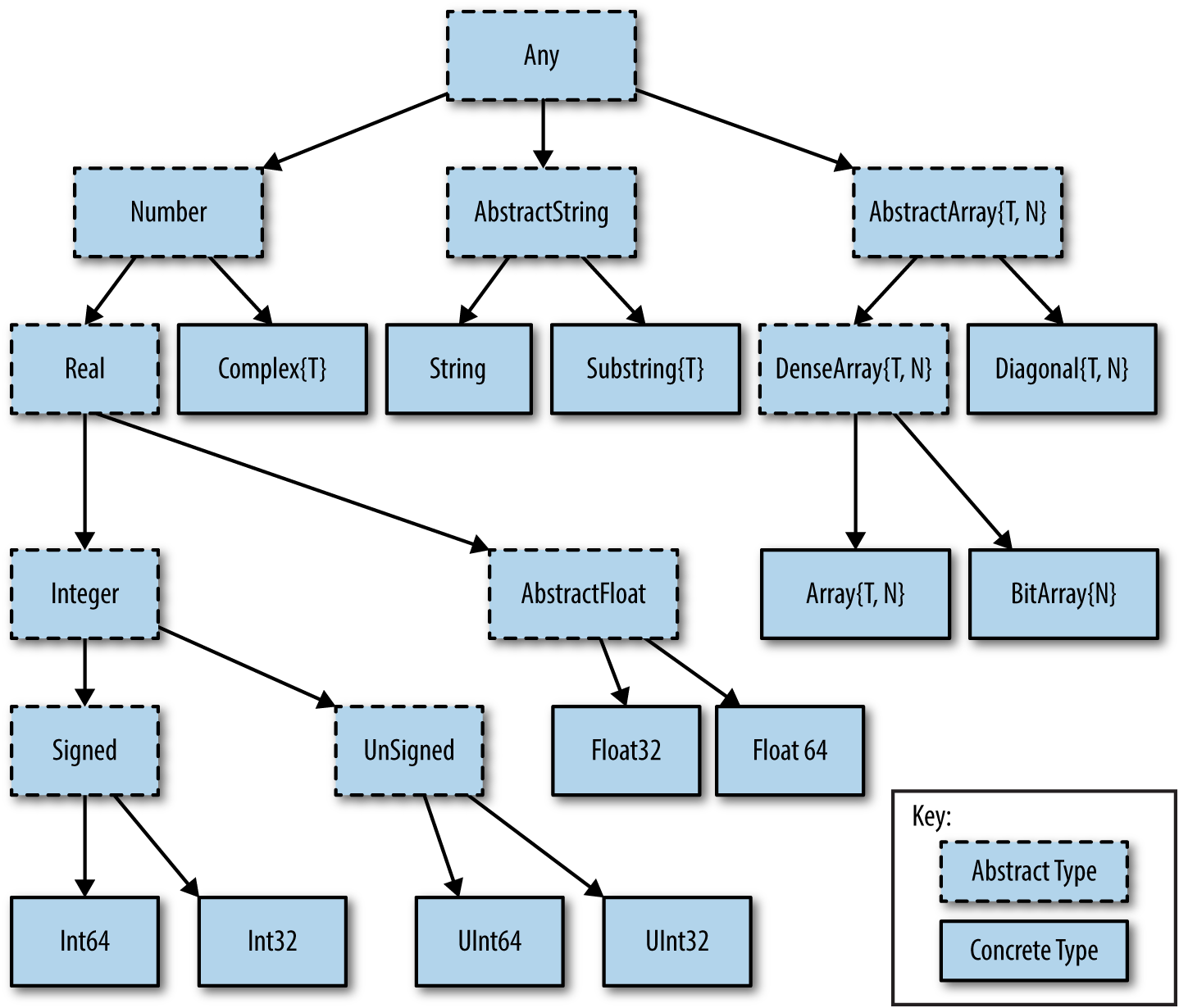# Types

Author

Marie-Hélène Burle

## Types systems

### Two main type systems in programming languages

#### Static type-checking

Type safety (catching errors of inadequate type) performed at compilation time.

Examples: C, C++, Java, Fortran, Haskell.

#### Dynamic type-checking

Type safety performed at runtime.

Examples: Python, JavaScript, PHP, Ruby, Lisp.

### Julia type system

Julia type system is dynamic (types are unknown until runtime), but types can be declared, optionally bringing the advantages of static type systems.

This gives users the freedom to choose between an easy and convenient language, or a clearer, faster, and more robust one (or a combination of the two).

## Julia types: a hierarchical tree

At the bottom:  concrete types.
Above:     abstract types (concepts for collections of concrete types).
At the top:     the `Any` type, encompassing all types.From O’Reilly

One common type missing in this diagram is the boolean type.

It is a subtype of the integer type, as can be tested with the subtype operator `<:`

``Bool <: Integer``
``true``

It can also be made obvious by the following:

``false == 0``
``true``
``true == 1``
``true``
``````a = true;
b = false;
3a + 2b``````
``3``

## Optional type declaration

Done with `::`

``<value>::<type>``

Example:

``2::Int``
``2``

## Illustration of type safety

This works:

``2::Int``
``2``

This doesn’t work:

``2.0::Int``
``LoadError: TypeError: in typeassert, expected Int64, got a value of type Float64``

Type declaration is not yet supported on global variables; this is used in local contexts such as inside a function.

Example:

``````function floatsum(a, b)
(a + b)::Float64
end``````
``floatsum (generic function with 1 method)``

This works:

``floatsum(2.3, 1.0)``
``3.3``

This doesn’t work:

``floatsum(2, 4)``
``LoadError: TypeError: in typeassert, expected Float64, got a value of type Int64``

## Information and conversion

The `typeof` function gives the type of an object:

``typeof(2)``
``Int64``
``typeof(2.0)``
``Float64``
``typeof("Hello, World!")``
``String``
``typeof(true)``
``Bool``
``typeof((2, 4, 1.0, "test"))``
``Tuple{Int64, Int64, Float64, String}``

Conversion between types is possible in some cases:

``Int(2.0)``
``2``
``typeof(Int(2.0))``
``Int64``
``Char(2.0)``
``'\x02': ASCII/Unicode U+0002 (category Cc: Other, control)``
``typeof(Char(2.0))``
``Char``

## Stylistic convention

The names of types start with a capital letter and camel case is used in multiple-word names.